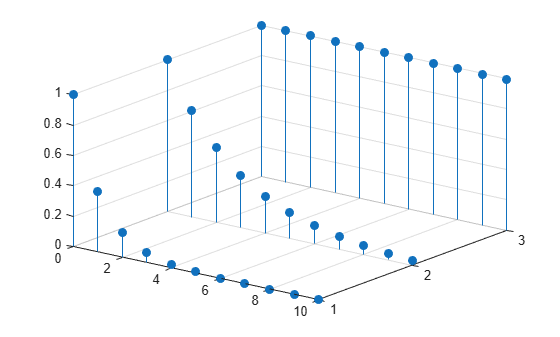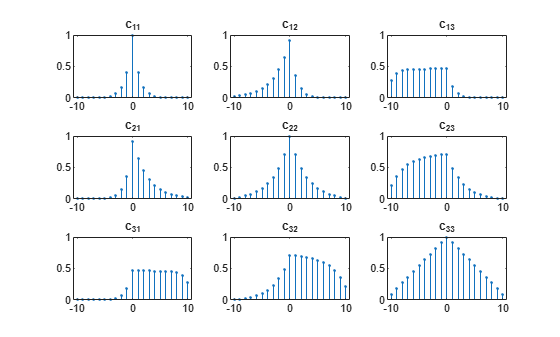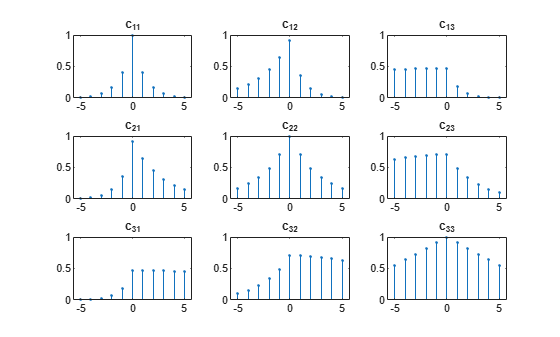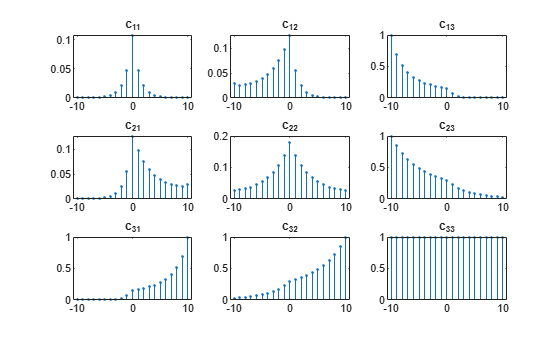# Cross-Correlation with Multichannel Input

Generate three 11-sample exponential sequences given by $0.{4}^{n}$, $0.{7}^{n}$, and $0.99{9}^{n}$, with $n\ge 0$. Use `stem3` to plot the sequences side by side.

```N = 11; n = (0:N-1)'; a = 0.4; b = 0.7; c = 0.999; xabc = [a.^n b.^n c.^n]; stem3(n,1:3,xabc','filled') ax = gca; ax.YTick = 1:3; view(37.5,30)```Compute the autocorrelations and mutual cross-correlations of the sequences. Output the lags so you do not have to keep track of them. Normalize the result so the autocorrelations have unit value at zero lag.

```[cr,lgs] = xcorr(xabc,'coeff'); for row = 1:3 for col = 1:3 nm = 3*(row-1)+col; subplot(3,3,nm) stem(lgs,cr(:,nm),'.') title(sprintf('c_{%d%d}',row,col)) ylim([0 1]) end end```Restrict the calculation to lags between $-5$ and $5$.

```[cr,lgs] = xcorr(xabc,5,'coeff'); for row = 1:3 for col = 1:3 nm = 3*(row-1)+col; subplot(3,3,nm) stem(lgs,cr(:,nm),'.') title(sprintf('c_{%d%d}',row,col)) ylim([0 1]) end end```Compute unbiased estimates of the autocorrelations and mutual cross-correlations. By default, the lags run between $-\left(N-1\right)$ and $N-1$.

```cu = xcorr(xabc,'unbiased'); for row = 1:3 for col = 1:3 nm = 3*(row-1)+col; subplot(3,3,nm) stem(-(N-1):(N-1),cu(:,nm),'.') title(sprintf('c_{%d%d}',row,col)) end end```